# Heat Motility

The goal for our heat motility system is to obtain higher concentrations of silk in close proximity to the implant. This is achieved by immobilizing the silk producing bacteria once they are within some distance to the implant, which is enabled by integrating the standard chemotaxis system with the DesK membrane fluidity sensor system. The result is that Bacillus subtilis stops moving when it is 37°C, and swims when it is 25°C. In our model we simulate the behavior of these systems and, if necessary, implement biologically plausible modifications to make it feasible.

## The chemotaxis system of B. subtilis

We first setup a model for the standard chemotaxis system. This system enables bacteria to seek out areas of higher concentrations of nutrients by modifying the direction of flagella rotations as a response to changes in concentrations of attractants and repellents. An increase in the concentration of attractants results in more counter clockwise (CCW) rotations, and an increase in the concentration of repellents in more clockwise (CW) rotations, which corresponds to an increased chance of swimming straight and tumbling respectively. This effect is complemented by the systems essential ability to adapt to any concentration of attractant or repellent. Given a homogenous environment, the system will therefore always maintain the same swimming to tumbling ratios.

At the molecular level the flagella behavior is controlled directly by the concentration of phosphorylated CheY (YP): more YP results in more CCW rotations (more swimming) and less YP in more CW rotations (more tumbling). YP is controlled by phosphorylated CheA concentrations (AP), its natural decay rate, and by CheC_CheD complex concentrations (CD), which act as YP phosphorylases. Because the natural and CD induced YP decay rates are unknown, we combined them by making the k11 rate coefficient a function of CD with the natural YP decay rate as its offset.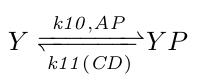Chemical reaction scheme 1.

In order to obtain proper swimming behavior, we require an initial increase and a delayed decrease in YP. The initial YP increase is due to an in increase in AP (which donate their phosphor groups to CheY) as a response to increased attractant concentrations. The decrease in YP is realized by two negative feedback systems (the CheV and the methylation subsystem), which decrease CheA phosphorylation rates, and by one negative feedforward system (the CheC-CheD subsystem), which increases the YP decay rate.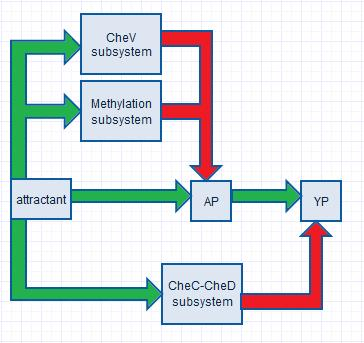Figure 1. The chemotaxis system of B.subtilis. The green arrows indicate a stimulatory function, and the red arrows an inhibitory function.

### CheA phosphorylation

Inside the cell CheW, CheA and CheV form complexes with the inner membrane receptor protein. When attractants bind to the receptor, CheA undergoes a change in conformation that enables it to be phosphorylated.

Following the example of David C. and John Ross , we assume the binding and dissociation of attractants with their receptors is fast, and that it can be described with the equilibrium equation (1), where KD is the dissociation constant for the aspartate receptor (which is focus of this model). The concentration of unbound receptors is then given by equation (2).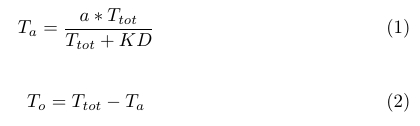Due to high concentrations of ATP in the cell, we assume similar kinetics for the CheA phosphorylation rate. It then follows that the positive increase in AP levels is proportional to bound receptor (Ta) and unbound receptor (T0) levels, and can be described by equation (3), where ATR is a constant.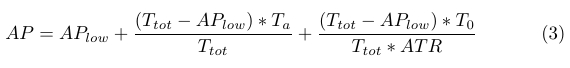### The CheV subsystem

The first adaption system of B.subtilis, the CheV subsystem, contributes to a delayed decrease in YP through a decrease in AP. This is realized by phosphorylated CheV (VP), which obtains its phosphor group from AP, after which it may disrupt the bond between the attractant-bound receptor and CheA, hereby inhibiting CheA phosphorylation .

Because AP levels are proportional to attractant levels we neglect the AP in our reactions (chem reaction 2) and take the rate coefficient k1 as a linear function of attractant levels. This disrupts the feedback loop between AP and VP, and makes the optimization later easier (see 'Parameter optimization' section).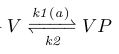Chemical reaction scheme 2

The negative decrease in AP due to VP is approximately 22% of the positive increase in AP described in equation (3), and is incorporated into the AP formula by subtraction in equation (4).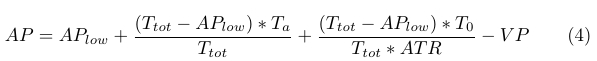### The methylation subsystem

The second adaption system of B.subtilis is the methylation system, which regulates the CheA phosphorylation rate through phosphorylated CheB (BP) and CheR. After obtaining its phosphor group from AP, BP can shuffle methyl groups between different receptor residues. It is thus proposed that the methylation of certain receptor residues can activate the receptor, whilst the methylation of others can deactivate it .

In order to provide negative AP feedback, BP would then shuffle methyl groups on the activation residues to the deactivation residues, whilst CheR shuffles methyl groups from the deactivation residues to the activation residues. We describe this system with the chemical reactions given below, where TM0 represents the methyl-deactivated receptors and TM1 the methyl-activated receptors.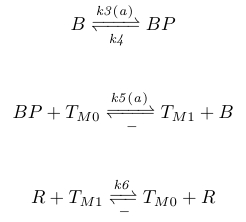Chemical reaction scheme 3.

Rate coefficients k3 and k5 are again linear functions of attractant concentrations, which enables us to eliminate the feedback loop involving AP, BP, and TM1, and is again justified by the fact that AP is proportional to attractant levels. The negative AP feedback involving TM1 is incorporated into equation (4) simply by subtracting TM1 from AP, and is given by equation (5). The total amount of negative AP feedback is approximately 35% of the positive increase.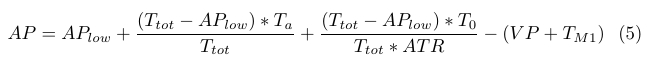### The CheC-CheD subsystem

The third adaption system of B.subtilis involves the CheC and CheD chemotaxis proteins. As of yet there appears to be no consensus on the exact behavior of this system. Christopher V. Rao. et al (2008) propose two viable options; a negative feedback and a negative feedforward system.

In the negative feedback system YP binds to CheC and CheD to form a complex. In doing so, it may pull receptor-bound CheD from the receptor where it functions as a stimulatory protein with respect to CheA phosphorylation. In this system, YP is therefore decreased through AP by means of removing CheD from the receptor

In the negative feedforward system CheD is also bound to the receptor, but it no longer has its stimulatory function. Instead, it is released from the receptor upon the binding of attractant, after which it may form a complex with CheC where it functions as a YP phosphatase.

Contrary to Christopher et al (2008), we propose that the negative feedforward system is the more likely case since the negative feedback system includes a time extensive feedback loop involving AP and YP through CheC, CheD, and the receptors. In our test simulations (not presented here), we observed adaption times of up to 10 minutes, whilst wild type B.subtilis adaption times are approximately one minute .

The chemical reactions for this negative feedforward system can be seen below, where TD is the concentration of receptors with CheD bound, T_ the concentration of receptors with no CheD bound, and C CheC. We furthermore model the receptors change in conformation, and the following CheD release, by taking k7 as a non-linear function of attractant levels with an offset equal to its CheD release rate with no attractant present.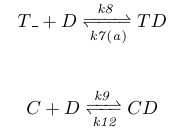Chemical reaction scheme 4.

### Parameter optimization

Although the rate coefficients and molecular concentrations of the chemotaxis system in E.coli are relatively well documented, for B.subtilis they are mostly unknown. Where possible, we therefore adopt values from the E.coli chemotaxis system, for which Li and Hazelbauer (2008) have measured the following molecular concentrations: CheA+AP=5.3µM, CheB+BP=0.28µM, CheY+YP=9.7µM, and CheR=0.16µM.

We now assume that CheA and CheV are saturated with respect to the formation of receptor complexes with CheW and the inner membrane receptor protein. We further assume that the conserved molecular concentrations of CheD are equivalent to CheA, so that we can state the following: CheV+VP = CheA+AP = Ta+T0 = TD+T_ = TM1+TM0 = CD+TD+D = 5.3µM

To find appropriate values for the rate coefficients we applied the simulated annealing algorithm. This is a heuristic-driven & probabilistic optimization algorithm that can be used to find optimal solutions for complex problems. In our case, we require the algorithm to find the set of parameters that enable exact adaption of YP concentrations.

Before we can apply the algorithm, we must define an error function. The error function determines what will be optimized, as it is the result of this function that the algorithm will strive to minimize. Because we are interested in the steady state value of YP, we require all molecular values to be in equilibrium before any further assessment can be established. The core of the error function for some set of parameters is therefore defined as the distance of each molecular concentration from its equilibrium value.

We first applied the algorithm to the CheV subsystem for attractant levels 0 and 5.8 (saturation). The error function was defined as the distance of each molecular concentration from its equilibrium value, along with the requirement that the total amount of negative AP feedback was 20 to 40% of the total attractant-induced AP increase. The same procedure was applied to the methylation subsystem, and, finally, to the system as a whole, where the previously optimized parameters were of course not further optimized. The error function was also modified to ensure that the YP equilibrium was constant across all levels of attractant ranging from 0 to 5.8.

We used matlabs ode45 function to simulate the differential equations for different levels of attractant. The simulations show how YP reactes to the addition and removal of different levels of attractant, which correspond to B.subtilis swimming and tumbling along increasing and decreasing gradients of attractant respectively. The tumbling along the decreasing gradients ensures that the bacteria will not swim deeply into undesirable areas, but will instead tumble at the edge until adaption has taken place, and it resumes its standard run and tumble behavior. Because it is still on the edge of the more desirable region it will have a higher chance of falling back in, after which YP will again increase so that B.subtilis can swim deeper into the nutrient rich area.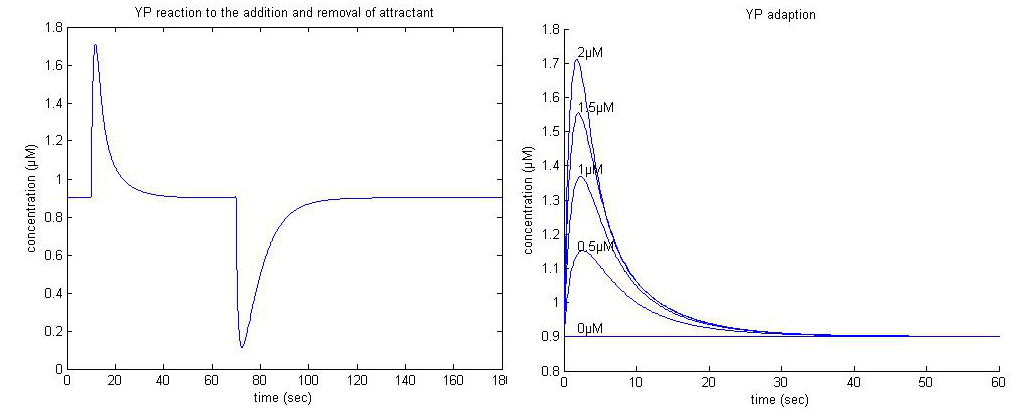Figure 2. Left: YP adaption to the addition and removal of 2µM attractant at t=10 and t=70 respectively. Right: YP adaption to different concentrations of attractant.

## Motility model

Before we can simulate the swimming behaviour of B.subtilis we need to relate YP to flagella rotations, for which we use the formula provided by Kuo and Koshland (1989), seen in equation (6), where h is a constant we use to fit our steady state YP value to data. P(CCW) can then be calculated by 1-P(CW).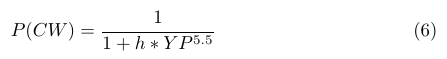We know that in normal steady state conditions the chance of flagella spinning CCW is 0.65, so that P(CCW) = 0.65 and P(CW)=0.35 . We also know that the mean swimming period is ~1.02 seconds and the mean tumbling period 0.165 seconds , so that for every 100 ms the chance of swimming given a swimming state is 0.903%, and the chance of tumbling given a tumbling state is 0.6061, which is to say: P(S|S)= 0.903 and P(T|T)= 0.6061. We also know that when CCW=0, that P(S|S)=1 and P(T|T)=0, and that when CCW=1, that P(S|S)=0 and P(T|T)=1. We can now use these points to fit two curves (equations 7 and 8), which can provide the threshold for switching from a tumbling state to a swimming state, and vica versa. The curves and equations are given below as a function of P(CCW).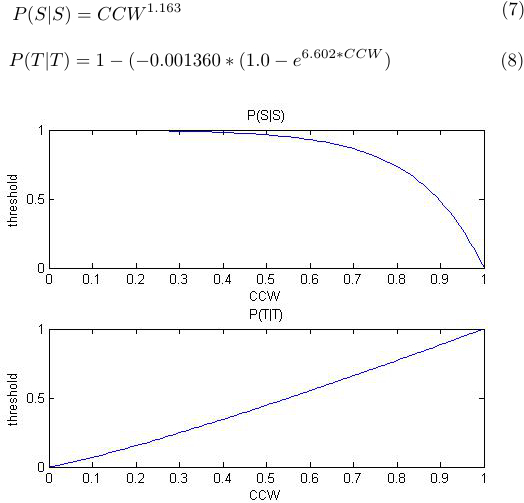Figure 3. Top: the probability of continuing a swim every 100 ms as a function of flagella CCW rotations. Bottom: the probability of continuing a tumble every 100 ms as a function of flagella CCW rotations.

In order to test whether our model demonstrates proper chemotaxis behavior, we simulate B.subtilis swimming in a small container with attractants. In the first simulation the dimensions of the container are approximately 250 by 250 micrometers, and the simulation is speeded up to 4x real time. This simulation shows how B.subtilis prefers to swim along increasing gradients of attractant, and tumble along decreasing gradients of attractant. In the second simulation the container is approximately 500 by 500 micrometers, and it is speeded up to 8x real time. Here the bacteria require more time to find the attractant, but when they do they get 'trapped' inside the regions with higher attractant concentrations.

 Simulations 1 and 2. Left; chemotaxis behaviour of B.subtilis in a ~250 by ~250 micrometer container in 4x real time. Right; chemotaxis behaviour of B.subtilis in a ~500 by ~500 micrometer container in 8x real time.

### Modifications

After observing the swimming behavior of B.subtilis in homogenous and non-homogenous environments, we realized that too much time would be required for Bacillus to swim from the cold to the warm region: in a homogenous environment the swimming behavior is too random, and in a non-homogenous environment it may get trapped in regions with higher concentrations of attractant, and otherwise its progress will get stalled by decreasing gradients of attractants.

A solution is to knockout one of the adaption systems to increase YP levels . This then increase P(CCW), and hence the chance of maintaining a swimming state. We simulate a CheC knockout, and plot the traversed path of 10 bacteria with and without the knockout (figure 4).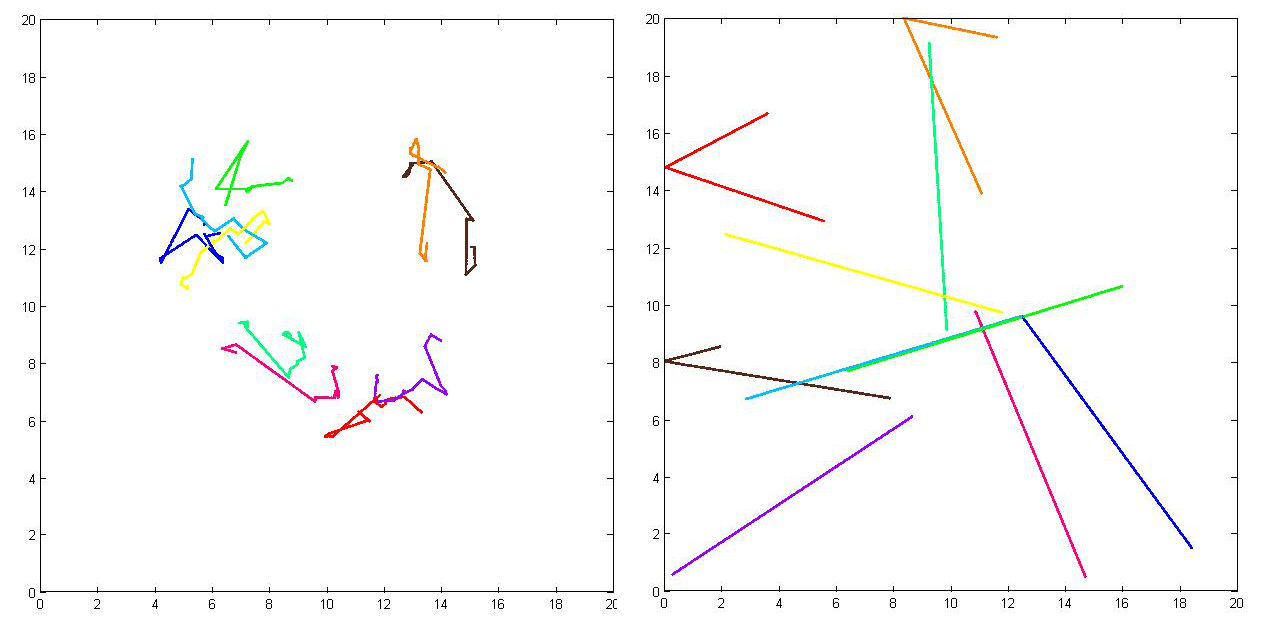Figure 4. Left: traversed pathway of 10 B.subtilis bacteria in an environment with no attractant. Right: traversed pathway of 10 B>subtilis bacteria with a CheC knockout in an environment with no attractant.

The animation below shows how B.subtilis cheC null mutants move around in an environment with attractants. It no longer cares if its swimming up a gradient of increasing attractants, or down a gradient of decreasing attractants.

 Simulation 3. The chemotaxis behaviour of a cheC B.subtilis null mutant in a ~250 by ~250 micrometer container in 4x real time.

## The effects of temperature

The temperature range in which B.subtilis will be operating in is 25°C to 37°C. We therefore require our model to be valid within this range. To test this, we use a general rule of thumb, which states that rate coefficients approximately double with every 10°C increase. We use equation (9), which is valid for temperatures ranging from 25°C to 47°C, and where k is the rate coefficient in question, T the temperature and k' the new rate coefficient.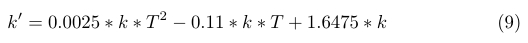In the literature, YP adaption has been observed for temperatures of up to 42°C . Adaption for 2 µM attractant for temperatures of 25°C, 31°C, and 37°C are plotted below (figure 5).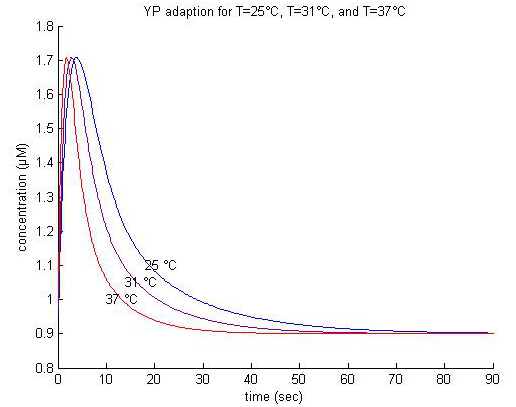Figure 5. The effect of temperature on YP adaption for 2 µM attractant.

### The DesK system

We are now ready to couple our modified chemotaxis system to the modified membrane fluidity sensor system DesK (read more about the DesK system here). In this system CheY is placed under the control of the des promoter so that CheY production is maximized at 25°C and minimized at 37°C. We thus hope for smooth swimming in the cold regions, and excessive tumbling in the warm regions.

Perhaps the most essential parameter for this process is the des promoter strength, which is, unfortunately, unknown. What we do know is that the number of PoPs at 25°C is 10 to 15 times higher than at 37°C, and that the decrease in temperature follows an exponential curve [8, 9]. We therefore assume that the promoter strength at 37°C is small enough to cause excessive tumbling, and that at 25°C CheY+YP is 10-15 times larger than at 37°C. The conserved molecular concentrations of CheY+YP are scaled with the temperature and can be seen below (figure 6).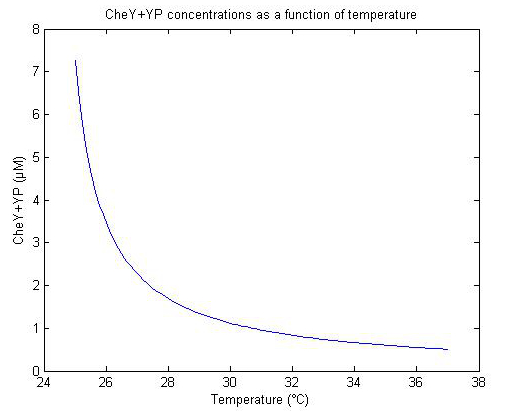Figure 6. Conserved CheY+YP concentrations as a function of temperature.

We now simulate the swimming behavior of 50 bacteria with modified chemotaxis and DesK systems. The container is approximately 500 by 500 micrometers and the temperature increases linearly with the y-axis from 25°C to 37°C.

The first simulation is 4x real time, and the bacteria start close to the 25°C region. We observe that the bacteria cant get past the ~30-32°C region due to excessive tumbling, and that the bacteria will therefore never reach the 37°C region.

The second simulation is 8x real time, and the bacteria now start in or close to the 37°C region. We see that our original concept is indeed valid, and that the bacteria should never escape the 37°C region due to excessive tumbling.

The third simulation is also 8x real time, but the initial positions of the bacteria are now random. We see that the system has very little effect on the concentration of bacteria throughout the container since the end distribution is also random.

 Simulations 4, 5, and 6. Left: simulation of 50 B.subtilis units in a container of approximately ~500 by ~500 micrometers with a linear temperature gradient ranging from 25°C to 37°C, and with the initial position of the bacteria located near the 25°C area. Middel: simulation of 50 B.subtilis units in a container of approximately ~500 by ~500 micrometers with a linear temperature gradient ranging from 25°C to 37°C, and with the initial position of the bacteria located near the 37°C area. Right: simulation of 50 B.subtilis units in a container of approximately ~500 by ~500 micrometers with a linear temperature gradient ranging from 25°C to 37°C, and where the initial position of the bacteria is random.

### Conclusion

We conclude that this system could potentially be used to keep bacteria away from warm regions. It is therefore not an optimal solution for obtaining a higher concentration of bacteria in close proximity to the implant (which is located in the 37°C region). To make this system somewhat feasible for our concept, we would have to carefully inject the bacteria directly into the 37°C region.

# Parameters

Protein concentrations
Value Reference
Y+YP 9.7µM 
B+BP 0.28µM 
CheR 0.16 
A+AP 5.3µM 
Ta+T0 5.3µM -
V+VP 5.3µM -
CheD+CD+TD 5.3µM -
TD+T_ 5.3µM -
TM0+TM1 5.3µM -
CheC+CD 3.9686µM Optimized value

Rate coefficients Value
k2 2.79937
k4 3.21494
k6 0.74553
k8 0.09981
k9 0.04779
k10 0.11346
k12 1.39741

Constants Value
k11_basal 0.08506
k11_scale 3.976
ATR 44.9309
KD 0.5
APlow 0.4949
h 0.9678

Dependent rate coefficients Function
k1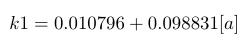k3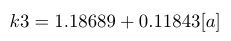k5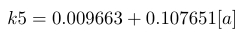k7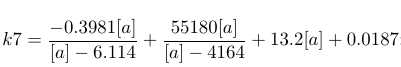k11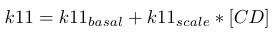# Ordinary differential equations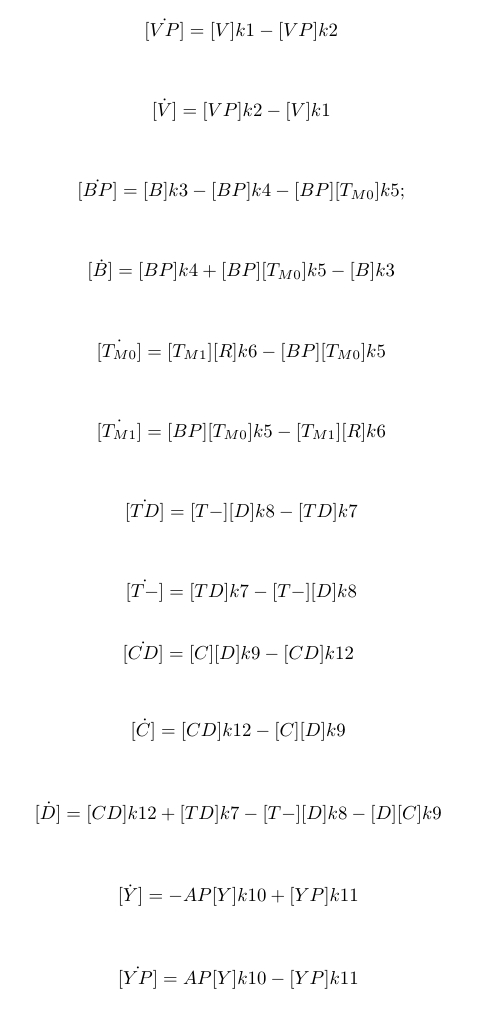# Matlab code

The code below contains the general chemotaxis model

The code below contains the general chemotaxis model where the temperature can be controlled

## References

 David C. Hauri and John Ross. (1995). A Model of Excitation and Adaptation in Bacterial Chemotaxis. Biophysical Journal Volume 68, pp708-722.

 Christopher V. Rao, George D. Glekas2 and George W. Ordal. (2008). Elsevier Ltd.

 Li, M. and G. L. Hazelbauer, “Cellular Stoichiometry of the Components of the Chemotaxis Signaling Complex,” J. Biol., 186, 3687–3694 (2004).

 Victor Sourjik and Howard C. Berg. (2001). Receptor sensitivity in bacterial chemotaxis. PNAS, vol 99. no 1. pp 123-127.

 Berg, H. C., and D. Brown. 1972.Chemotaxis in Escherichia coli analyzed by three dimensional tracking. Nature. 239:500-504.

 Ordal GW, Gibson KJ. (1977). Chemotaxis toward amino acids by Bacillus subtilis. J Bacteriol. vol 129(1):151-5.

 Rosario, M.L., Kirby, J., Bochar, D., and Ordal, G.W. (1995) Chemotactic methylation and behavior of Bacillus subtilis :role of two unique proteins, CheC and CheC. Biochemistry 34: 3823-3831.

 Thermal Regulation of Membrane Lipid Fluidity by a Two-Component System in Bacillus subtilis* Received for publication, January 3, 2011, and in revised form, January 31, 2011 BIOCHEMISTRY AND MOLECULAR BIOLOGY EDUCATION Vol. 39, No. 5, pp. 362–366, 2011

 Larisa E. Cybulski, Daniela Albanesi, Maria C. Mansilla, Silvia Altabe, Pablo S. Aguillar, & Diego de Mendoza. (2002). Mechanism of membrane fluidity optimization: isothermal control of the Bacillus subtilis acyl-lipid desaturase. Molecular microbiology. Vol 45(5). pp; 1379-1388